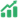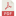# High School Students’ Generalization Viewed from Logical-Mathematical Intelligence

• Jihan Nurilla Universitas Negeri Surabaya
• Susanah Susanah

### Abstract

Generalization is an important element in understanding, recognizing, and examining mathematical situations. Students' generalization processes can be analyzed according to Mason's four stages of the generalization process, including the perception, expression, symbolic expression, and manipulation of generality. One of the things that may cause differences in students' generalization processes is logical-mathematical intelligence. This research aims to describe high school students' generalization processes in terms of logical-mathematical intelligence. This research is using a descriptive qualitative method. The subjects of this study consisted of 3 high school students in Surabaya with high, moderate, and low logical-mathematical intelligence. The data is obtained from the Logical-Mathematical Intelligence Questionnaire and Generalization Task. The material used in the Generalization Task is curved-face solid. The results of this study indicate that the student with low logical-mathematical intelligence has reached the stage of identifying patterns or regularity in the given information, but the student doesn't use those patterns to determine the next term. In other words, the student with low logical-mathematical intelligence has only reached the stage of perception of generality. The student with moderate logical-mathematical intelligence has reached the stage of identifying patterns or regularity in the given information and using those patterns to determine the next term but the student doesn’t reach the stage of formulating the general formula of the pattern that has been found. In other words, the student with moderate logical-mathematical intelligence has only reached the stage of perception and expression of generality. The student with high logical-mathematical intelligence has reached the stage of identifying patterns or regularity and used those patterns to determine the next term. In addition, the student with high logical-mathematical intelligence also reaches the stage of formulating general formulas of patterns that have been found and using the general formula to solve the given problem In other words, the student with high logical-mathematical intelligence has reached all the stages which is perception, expression, symbolic expression, and manipulation of generality.

Published
2022-01-19
Section
ArticlesAbstract View: 37PDF_111-120 Download: 41